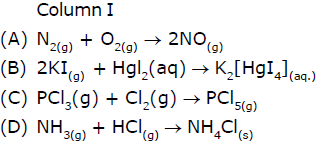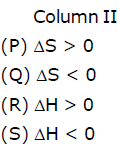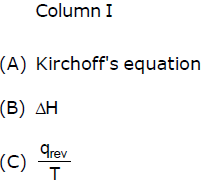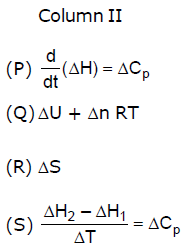Courses

25 Questions MCQ Test Chemistry for JEE Advanced | Thermochemistry MCQ - 1 (Advanced)

Description
Attempt Thermochemistry MCQ - 1 (Advanced) | 25 questions in 50 minutes | Mock test for JEE preparation | Free important questions MCQ to study Chemistry for JEE Advanced for JEE Exam | Download free PDF with solutions
*Multiple options can be correct
QUESTION: 1

Solution:
QUESTION: 2

Which of the following statements is / are correct with respect to Carbon Credits?  Certified Emission Reduction (CER) certificate is an titlement of Carbon Credits Transfer of carbon credits is taxable in India Select the correct option from the codes given below:

Solution:
*Multiple options can be correct
QUESTION: 3

Which is (are) correct for ideal gas ?

Solution:
*Multiple options can be correct
QUESTION: 4

Which of the following are applicable for a thermochemical equation ? It tells :

Solution:
*Multiple options can be correct
QUESTION: 5

The heat of reaction depends upon :

Solution:
*Multiple options can be correct
QUESTION: 6

The standard heat of formation of a compound is the :

Solution:
*Multiple options can be correct
QUESTION: 7

The heat of neutralization of a strong acid by a strong base is a constant because :

Solution:
*Multiple options can be correct
QUESTION: 8

Which of the following statements is (are) true ?

Solution:
*Multiple options can be correct
QUESTION: 9

The standard heat of formation of U3O8 is -853.5 kcal mol-1 and standard heat of the reaction, 3UO2 + O2 → U3O8 is -76.01 kcal. The standard heat of formation of UO2 is (are) :

Solution:
*Multiple options can be correct
QUESTION: 10

For which of the following substances is the heat of formation in the standard state zero ?

Solution:
*Multiple options can be correct
QUESTION: 11

Which of the following statements is (are) true ?

Solution:
*Multiple options can be correct
QUESTION: 12

The heat of combustion of ethanol was determined in a bomb calorimeter and was found to be -670.48 kcal mol-1 at 25°C. What will be DU for the same reaction at 298 K ?

Solution:
QUESTION: 13

Match the columnSolution:
QUESTION: 14

Match the columnSolution:
QUESTION: 15

Heat of reaction is the change in enthalpy or internal energy as represented by a balanced thermochemical
equation. The amount of energy released during a chemical change depends upon the state of reactants
and products, the conditions of pressure and volume at which reaction is carried out, and temperature.
The variation of heat of reaction (ΔH or ΔE) with temperature is given as ΔH2 – ΔH1 = ΔCp [T2 – T1] or ΔE2
– ΔE1 = ΔCv (T2 – T1). Standard heat enthalpy of elements in their most stable state is assumed to be zero
whereas standard heat enthalpy of compound is referred as heat of formation of that compound at 1 atm
pressure and 25°C. Oxidation of N2 to N2O, NO, NO2 shows absorption of energy whereas heat of combustion
of N2 is exothermic like other heat of combustion.

Q. Standard heat enthalpy has been assumed to be zero for :

Solution:
QUESTION: 16

Heat of reaction is the change in enthalpy or internal energy as represented by a balanced thermochemical
equation. The amount of energy released during a chemical change depends upon the state of reactants
and products, the conditions of pressure and volume at which reaction is carried out, and temperature.
The variation of heat of reaction (ΔH or ΔE) with temperature is given as ΔH2 – ΔH1 = ΔCp [T2 – T1] or ΔE2
– ΔE1 = ΔCv (T2 – T1). Standard heat enthalpy of elements in their most stable state is assumed to be zero
whereas standard heat enthalpy of compound is referred as heat of formation of that compound at 1 atm
pressure and 25°C. Oxidation of N2 to N2O, NO, NO2 shows absorption of energy whereas heat of combustion
of N2 is exothermic like other heat of combustion.

Q. Which statements regarding the formation of NO and NO2 respectively from N2 and O2 are correct:

(A) Heat of formation of NO is exothermic

(B) Heat of formation of NO2 is exothermic

(C) The oxidation of N2 to NO and NO2 is favoured at high temperature.

(D) If heat of formation of NO and NO2 are 21.55 and 8.50 kcal, heat of reaction for

NO + ½O2 → NO2 is -13.05 kcal

Solution:
QUESTION: 17

Heat of reaction is the change in enthalpy or internal energy as represented by a balanced thermochemical
equation. The amount of energy released during a chemical change depends upon the state of reactants
and products, the conditions of pressure and volume at which reaction is carried out, and temperature.
The variation of heat of reaction (ΔH or ΔE) with temperature is given as ΔH2 – ΔH1 = ΔCp [T2 – T1] or ΔE2
– ΔE1 = ΔCv (T2 – T1). Standard heat enthalpy of elements in their most stable state is assumed to be zero
whereas standard heat enthalpy of compound is referred as heat of formation of that compound at 1 atm
pressure and 25°C. Oxidation of N2 to N2O, NO, NO2 shows absorption of energy whereas heat of combustion
of N2 is exothermic like other heat of combustion.

Q. Heat of combustion of carbon in diamond and amorphous form are -94.3 and -97.6 kcal/mole. The heat required to convert 6 g carbon from diamond to amorphous form is :

Solution:
QUESTION: 18

Heat of reaction is the change in enthalpy or internal energy as represented by a balanced thermochemical
equation. The amount of energy released during a chemical change depends upon the state of reactants
and products, the conditions of pressure and volume at which reaction is carried out, and temperature.
The variation of heat of reaction (ΔH or ΔE) with temperature is given as ΔH2 – ΔH1 = ΔCp [T2 – T1] or ΔE2
– ΔE1 = ΔCv (T2 – T1). Standard heat enthalpy of elements in their most stable state is assumed to be zero
whereas standard heat enthalpy of compound is referred as heat of formation of that compound at 1 atm
pressure and 25°C. Oxidation of N2 to N2O, NO, NO2 shows absorption of energy whereas heat of combustion
of N2 is exothermic like other heat of combustion.

Q. Heat of vaporisation of H2O is 627.78 cal/g. If heat of formation of H2O(l) is  -68.3 kcal, heat of formation of H2O(g) is :

Solution:
QUESTION: 19

Heat of reaction is the change in enthalpy or internal energy as represented by a balanced thermochemical
equation. The amount of energy released during a chemical change depends upon the state of reactants
and products, the conditions of pressure and volume at which reaction is carried out, and temperature.
The variation of heat of reaction (ΔH or ΔE) with temperature is given as ΔH2 – ΔH1 = ΔCp [T2 – T1] or ΔE2
– ΔE1 = ΔCv (T2 – T1). Standard heat enthalpy of elements in their most stable state is assumed to be zero
whereas standard heat enthalpy of compound is referred as heat of formation of that compound at 1 atm
pressure and 25°C. Oxidation of N2 to N2O, NO, NO2 shows absorption of energy whereas heat of combustion
of N2 is exothermic like other heat of combustion.

The specific heat of I2 in vapour and solid state are 0.031 and 0.055 cal/g-°C respectively. If heat of sublimation of iodine is 6.096 kcal mol-1 at 200°C, the heat of sublimation of I2 at 250°C is :

Solution:
QUESTION: 20

Heat of neutralisation is amount of heat evolved or absorbed when 1 g-equivalent of an acid reacts with
1 g-equivalent of a base in dilute solution. If weak acid or weak base are neutralised, the heat released
during neutralisation is some what lesser than -13.7 kcal or -57.27 kJ. Heat of neutralisation is also referred
as heat of formation of water from H+ and OH- ions i.e.,

H+ + OH- → H2O ; ΔH = -13.7 kcal.

Q. Heat of neutralisation of HF and acetic acid respectively are (in kcal) :

Solution:
QUESTION: 21

Heat of neutralisation is amount of heat evolved or absorbed when 1 g-equivalent of an acid reacts with
1 g-equivalent of a base in dilute solution. If weak acid or weak base are neutralised, the heat released
during neutralisation is some what lesser than -13.7 kcal or -57.27 kJ. Heat of neutralisation is also referred
as heat of formation of water from H+ and OH- ions i.e.,

H+ + OH- → H2O ; ΔH = -13.7 kcal.

Q. Which of the following statements are correct :

(1) ΔH = ΔU + ΔnRT

(2) Heat changes measured by bomb calorimeter give change in heat enthalpy during the reaction.

(3) ΔH = ΔU for the reaction : C(s) + O2(g) → CO2(g)

(4) Heat of formation for C6H6 can be directly measured

Solution:
QUESTION: 22

Heat of neutralisation is amount of heat evolved or absorbed when 1 g-equivalent of an acid reacts with
1 g-equivalent of a base in dilute solution. If weak acid or weak base are neutralised, the heat released
during neutralisation is some what lesser than -13.7 kcal or -57.27 kJ. Heat of neutralisation is also referred
as heat of formation of water from H+ and OH- ions i.e.,

H+ + OH- → H2O ; ΔH = -13.7 kcal.

Q. 200 mL of 0.1 M NaOH is mixed with 100 mL of 0.1 M H2SO4 in 1 experiment. In II experiment 100 mL of 0.1 M NaOH is mixed with 50 mL of 0.1 M H2SO4. Select the correct statements :

(1) Heat liberated in each of the two reactions is 274 cal.

(2) Heat liberated in I is 274 cal and in II is 137 cal

(3) Temperature rise in I reaction is more than the temperature rise in II.

(4) Temperature rise in I reaction is equal to the temperature rise in II.

Solution:
QUESTION: 23

Hess's law of heat of summation is based on law of conservation of energy. It has found significance in
deriving heats of many reactions which either do not take place or if take place, than other side reactions
also accompany it. For example heat of reaction for C + ½ O2 → CO can not be studied directly as it occurs
with simultaneous reaction of oxidation of CO to CO2. Bond energy is referred as heat enthalpy when a bond
is broken to produce isolated gaseous atoms. In case of breaking up of bonds between unlike atoms e.g.,
C – H bond in CH4, bond energy is referred as average bond energy as four C – H bonds are broken up.

Q. Which of the following statements are correct :

(1) The dissociation of bond is always endothermic.

(2) The formation of a bond is always exothermic.

(3) Heat of formation of an atom = ½ × bond energy like atoms covalent bond

(4) The heat enthalpy change in a chemical reaction is equal but opposite to the heat enthalpy if reaction is reversed.

(5) Hess's law can be verified experimentally.

Solution:
QUESTION: 24

Hess's law of heat of summation is based on law of conservation of energy. It has found significance in
deriving heats of many reactions which either do not take place or if take place, than other side reactions
also accompany it. For example heat of reaction for C + ½ O2 → CO can not be studied directly as it occurs
with simultaneous reaction of oxidation of CO to CO2. Bond energy is referred as heat enthalpy when a bond
is broken to produce isolated gaseous atoms. In case of breaking up of bonds between unlike atoms e.g.,
C – H bond in CH4, bond energy is referred as average bond energy as four C – H bonds are broken up.

Q. Heat of dissociation of CH4 and C2H6 are 360 and 620 kcal mol-1. The C - C bond energy would be :

Solution:
QUESTION: 25

Hess's law of heat of summation is based on law of conservation of energy. It has found significance in
deriving heats of many reactions which either do not take place or if take place, than other side reactions
also accompany it. For example heat of reaction for C + ½ O2 → CO can not be studied directly as it occurs
with simultaneous reaction of oxidation of CO to CO2. Bond energy is referred as heat enthalpy when a bond
is broken to produce isolated gaseous atoms. In case of breaking up of bonds between unlike atoms e.g.,
C – H bond in CH4, bond energy is referred as average bond energy as four C - H bonds are broken up.

Q.  If C + ½ O2 → CO(g) ; ΔH = – 110 kJ.
C + H2O(g) → CO(g) + H2(g) ; ΔH = 132 kJ

The mole composition of mixture of steam and O2 and steam on being passed over coke at 1273 K so that temperature remains constant is :

Solution:Use Code STAYHOME200 and get INR 200 additional OFF Use Coupon Code

Track your progress, build streaks, highlight & save important lessons and more!

Similar ContentRelated tests